table.module-2{width:98.87%;padding:0}table div table+table+table div table{width:98.87%;float:none;margin-left:auto;margin-right:auto;padding:0}table div table+table+table div table a{border:0 none;text-decoration:none}table div table+table+table div table img{width:100%!important;border:0 none;text-decoration:none}table div table+table+table div table td{width:100%;padding:0}/* styles */

## Christmas Holiday Ensembles at Kahala Mall

 table div table+table+table+table+table div table td,table.module-4{width:100%;padding:0}table div table+table+table+table+table div table{width:100%;float:none;margin-left:auto;margin-right:auto;padding:0}table div table+table+table+table+table div table a{border:0 none;text-decoration:none}table div table+table+table+table+table div table img{width:100%!important;border:0 none;text-decoration:none}/* styles */
 /* styles */ The Holiday Season is in full swing as string, piano and voice students from the Masaki School of Music prepare for their first performance this Friday, December 15th, at Kahala Mall. This 5:00pm performance is free and will be located indoors, next to Starbucks.
 table div table+table+table+table+table+table+table div table{width:100%;padding:0}table div table+table+table+table+table+table+table div table img{width:96.23%;padding:0;float:none}table div table+table+table+table+table+table+table div table td{width:100%;padding:0 1.88% 18px}/* styles */table div table+table+table+table+table+table+table+table div table,table.module-7{width:71.7%;float:left;padding:0}table div table+table+table+table+table+table+table+table div table a{border:0 none;text-decoration:none}table div table+table+table+table+table+table+table+table div table img{width:100%!important;border:0 none;text-decoration:none}table div table+table+table+table+table+table+table+table div table td{width:100%;padding:0 20px 20px 0}/* styles */ The residents at the Plaza at Waikiki will be treated to our Christmas Strings on December 20th. This is our way of sharing the wonderful Gift of Music.
 table div table+table+table+table+table+table+table+table+table div table{width:100%;padding:0}table div table+table+table+table+table+table+table+table+table div table img{width:96.23%;padding:0;float:none}table div table+table+table+table+table+table+table+table+table div table td{width:100%;padding:0 1.88% 18px}/* styles */## 35th Ala Moana CenterStage Christmas Extravaganza

 table div table+table+table+table+table+table+table+table+table+table+table div table{width:100%;padding:0}table div table+table+table+table+table+table+table+table+table+table+table div table table{padding:0;float:left!important;width:51.886%!important}table div table+table+table+table+table+table+table+table+table+table+table div table table+table td,table div table+table+table+table+table+table+table+table+table+table+table div table td{padding-left:0;padding-right:0}table div table+table+table+table+table+table+table+table+table+table+table div table table td{padding-left:0;padding-right:20px}table div table+table+table+table+table+table+table+table+table+table+table div table table+table{float:left!important;width:48.114%!important}/* styles */
 /* styles */ Masaki School of Music students have been rehearsing every weekend, preparing for our 35th Ala Moana Christmas Extravaganza Concert on Centerstage, Sunday, December 17, 2017 at 7:00pm. 125 piano students will perform traditional Holiday selections on 12 digital pianos. This concert is free and open to the public. We hope to see you there!
 table div table+table+table+table+table+table+table+table+table+table+table+table+table div table{width:100%;padding:0}table div table+table+table+table+table+table+table+table+table+table+table+table+table div table img{width:96.23%;padding:0;float:none}table div table+table+table+table+table+table+table+table+table+table+table+table+table div table td{width:100%;padding:0 1.88% 18px}/* styles */table.module-13{width:98.49%;padding:0}table div table+table+table+table+table+table+table+table+table+table+table+table+table+table div table{width:98.49%;float:none;margin-left:auto;margin-right:auto;padding:0}table div table+table+table+table+table+table+table+table+table+table+table+table+table+table div table a{border:0 none;text-decoration:none}table div table+table+table+table+table+table+table+table+table+table+table+table+table+table div table img{width:100%!important;border:0 none;text-decoration:none}table div table+table+table+table+table+table+table+table+table+table+table+table+table+table div table td{width:100%;padding:0}/* styles */
 /* styles */ The HMTA (Hawaii Music Teachers Association) will be featuring all the Hawaii winners in a concert at the UH Orvis Auditorium on Saturday, December 30th at 4:00pm. Noe Baladad will be one of the performers. To purchase the \$20 adult tickets (students are free), contact Noe at noebaladad@gmail.com or call her at (808) 341-8275.
 table div table+table+table+table+table+table+table+table+table+table+table+table+table+table+table+table div table{width:100%;padding:0}table div table+table+table+table+table+table+table+table+table+table+table+table+table+table+table+table div table img{width:96.23%;padding:0;float:none}table div table+table+table+table+table+table+table+table+table+table+table+table+table+table+table+table div table td{width:100%;padding:0 1.88% 18px}/* styles */## 2 out of 6 states represented by Masaki School of Music alumni!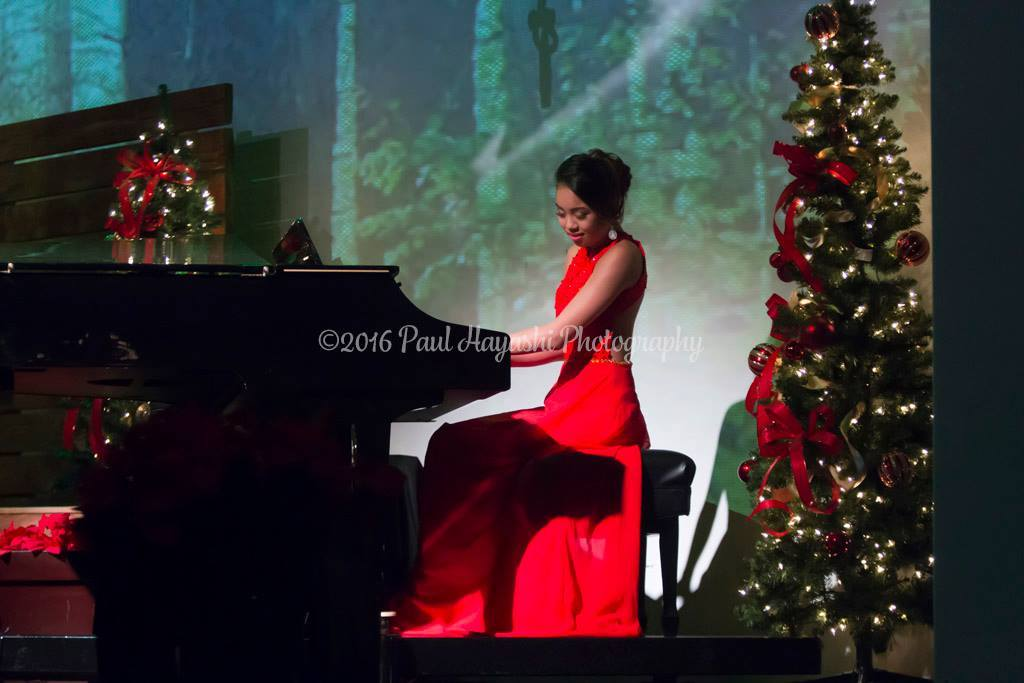Noe Baladad (Photo credit: Paul Hayashi Photography)
 /* styles */ Tyler Ramos and Noe Baladad, both former students of Ellen Masaki and, since 2010 students of Dr. Tommy Yee at the University of Hawaii, are heading to Las Vegas for the MTNA (Music Teachers National Association) Southwest Division's Young Artist Competition in January. Noe will represent Hawaii and Tyler, who just moved to Tucson, AZ to pursue his masters degree with Dr. John Milbauer at the University of Arizona, won AZ last month.
 table div table+table+table+table+table+table+table+table+table+table+table+table+table+table+table+table+table+table+table+table div table{width:100%;padding:0}table div table+table+table+table+table+table+table+table+table+table+table+table+table+table+table+table+table+table+table+table div table img{width:96.23%;padding:0;float:none}table div table+table+table+table+table+table+table+table+table+table+table+table+table+table+table+table+table+table+table+table div table td{width:100%;padding:0 1.88% 18px}/* styles */## Sing, Sing, Sing!

 /* styles */ We are proud to introduce our 2 new voice instructors, Sharon Myung and Brooke Alcuran. Besides teaching privately, they are ready to form a Masaki School of Music chorus! Many of our students have listed voice as their 2nd instrument of choice. Now is the time to step up and be a part of the first MSM Vocal Ensemble. Whether you would like to sing popular, folk, Hawaiian, classical, show tunes or inspirational songs, this is your opportunity to develop your singing voice while working with 2 highly trained singers in a group setting. If interested, email masakischoolofmusic@gmail.com or call 593-9395.
 table div table+table+table+table+table+table+table+table+table+table+table+table+table+table+table+table+table+table+table+table+table+table+table+table div table{width:100%;padding:0}table div table+table+table+table+table+table+table+table+table+table+table+table+table+table+table+table+table+table+table+table+table+table+table+table div table img{width:96.23%;padding:0;float:none}table div table+table+table+table+table+table+table+table+table+table+table+table+table+table+table+table+table+table+table+table+table+table+table+table div table td{width:100%;padding:0 1.88% 18px}/* styles */## Yamaha digital pianos for sale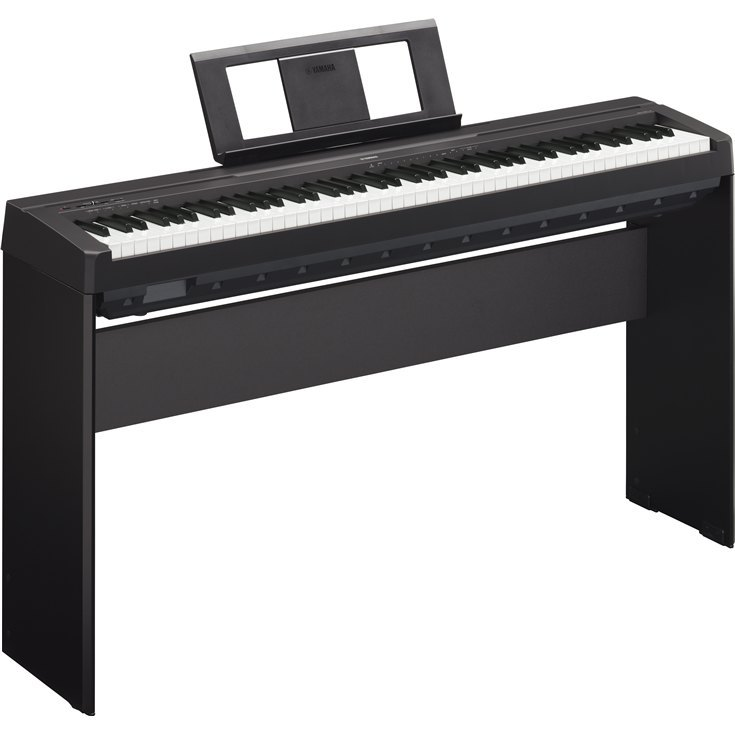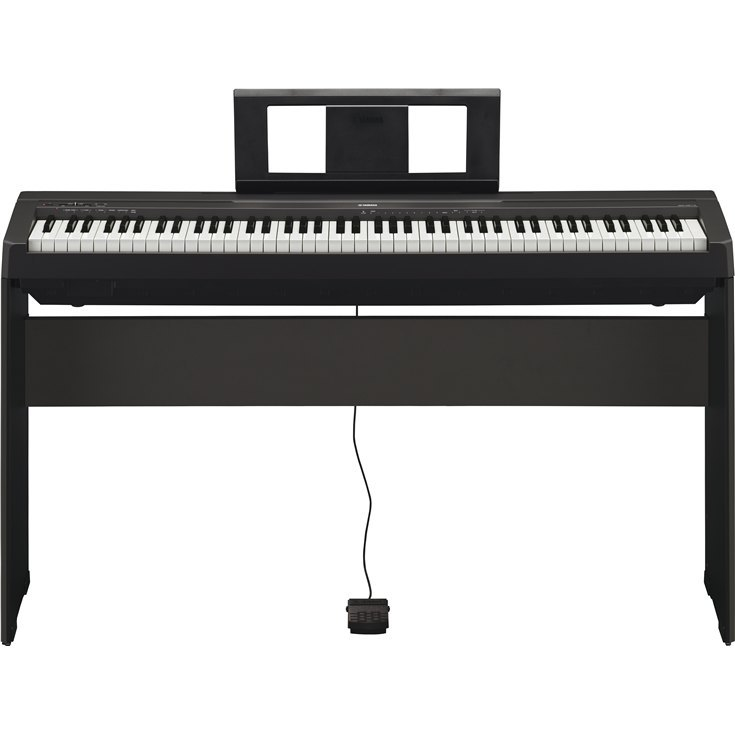/* styles */ The digital pianos that the students will be performing on at Ala Moana Centerstage will be on sale after the concert. There will be four Yamaha P45, 88-key weighted action digital pianos (as seen in photos above), selling for \$595 and includes a bench. Please contact Nancy Masaki at masakischoolofmusic@gmail.com to secure one for your young pianist!
 table div table+table+table+table+table+table+table+table+table+table+table+table+table+table+table+table+table+table+table+table+table+table+table+table+table+table+table+table div table{width:100%;padding:0}table div table+table+table+table+table+table+table+table+table+table+table+table+table+table+table+table+table+table+table+table+table+table+table+table+table+table+table+table div table img{width:96.23%;padding:0;float:none}table div table+table+table+table+table+table+table+table+table+table+table+table+table+table+table+table+table+table+table+table+table+table+table+table+table+table+table+table div table td{width:100%;padding:0 1.88% 18px}/* styles */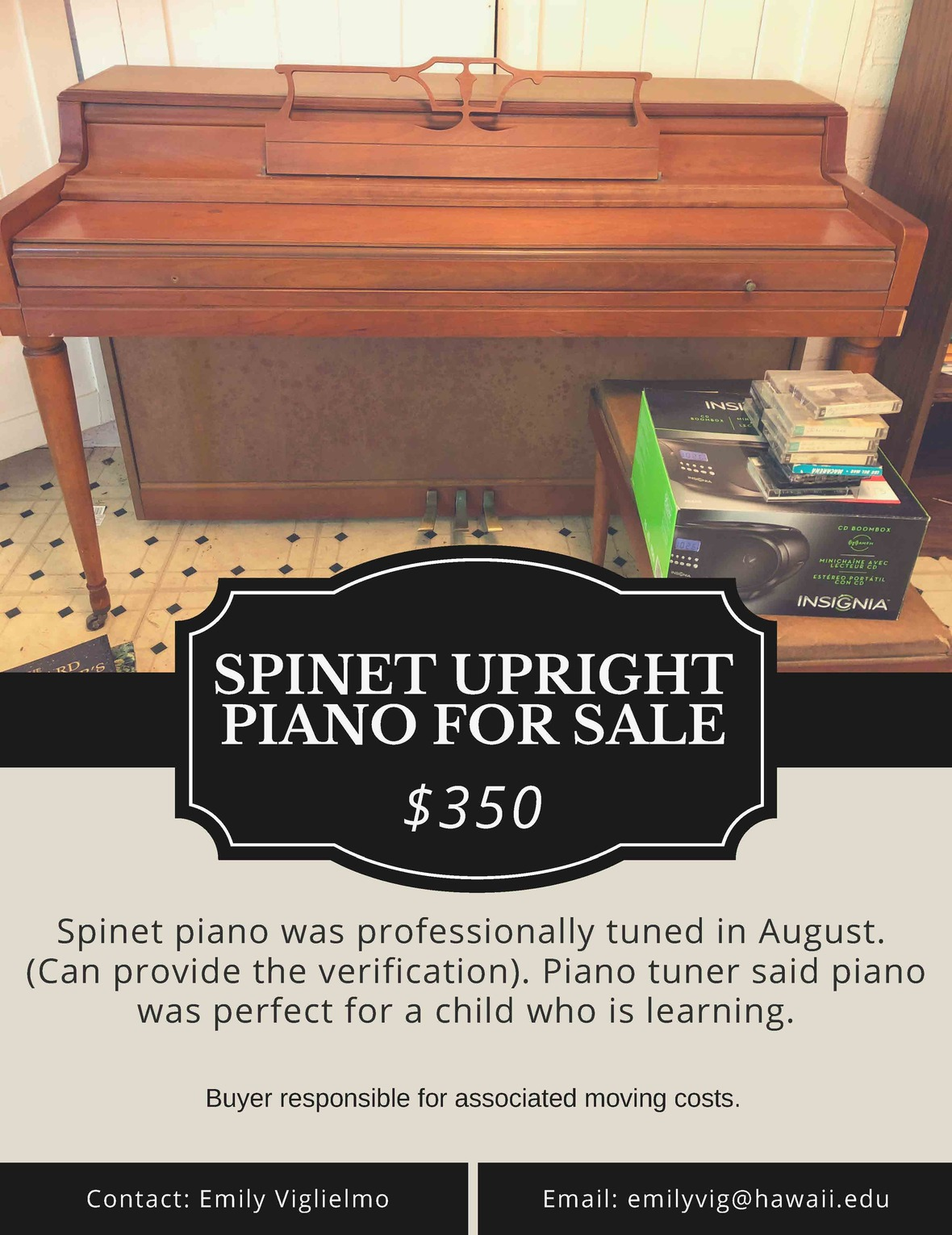## Piano for Sale

Contact Emily Viglielmo at emilyvig@hawaii.edu if you are interested.

 table div table+table+table+table+table+table+table+table+table+table+table+table+table+table+table+table+table+table+table+table+table+table+table+table+table+table+table+table+table+table div table{width:100%;padding:0}table div table+table+table+table+table+table+table+table+table+table+table+table+table+table+table+table+table+table+table+table+table+table+table+table+table+table+table+table+table+table div table img{width:96.23%;padding:0;float:none}table div table+table+table+table+table+table+table+table+table+table+table+table+table+table+table+table+table+table+table+table+table+table+table+table+table+table+table+table+table+table div table td{width:100%;padding:0 1.88% 18px}/* styles */## Gift Certificate for the Holidays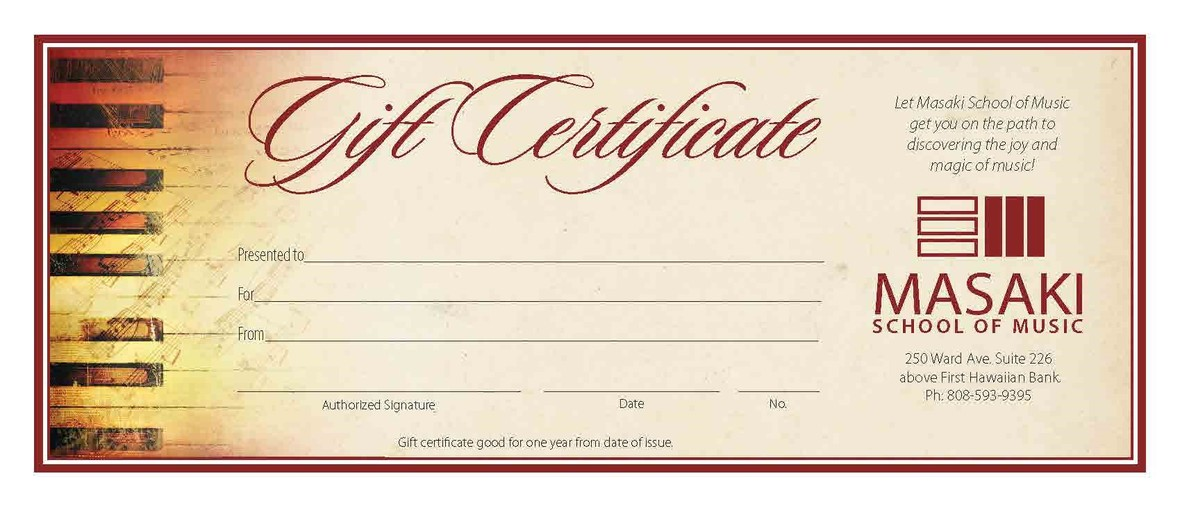Gift Certificates for music lessons (piano, violin, viola, cello, voice, guitar) make for the perfect Christmas Gift. Music is a gift that keeps on giving, so why not provide the incentive to get someone special on track for a lifetime of music.

Email: masakischoolofmusic@gmail.com or call (808) 593-9395 to order.

 table div table+table+table+table+table+table+table+table+table+table+table+table+table+table+table+table+table+table+table+table+table+table+table+table+table+table+table+table+table+table+table+table+table div table{width:100%;padding:0}table div table+table+table+table+table+table+table+table+table+table+table+table+table+table+table+table+table+table+table+table+table+table+table+table+table+table+table+table+table+table+table+table+table div table img{width:96.23%;padding:0;float:none}table div table+table+table+table+table+table+table+table+table+table+table+table+table+table+table+table+table+table+table+table+table+table+table+table+table+table+table+table+table+table+table+table+table div table td{width:100%;padding:0 1.88% 18px}/* styles */## Mele Kalikimaka me ka Hau'oli Makahiki Hou!

 table div table+table+table+table+table+table+table+table+table+table+table+table+table+table+table+table+table+table+table+table+table+table+table+table+table+table+table+table+table+table+table+table+table+table+table div table{width:100%;padding:0}table div table+table+table+table+table+table+table+table+table+table+table+table+table+table+table+table+table+table+table+table+table+table+table+table+table+table+table+table+table+table+table+table+table+table+table div table img{width:96.23%;padding:0;float:none}table div table+table+table+table+table+table+table+table+table+table+table+table+table+table+table+table+table+table+table+table+table+table+table+table+table+table+table+table+table+table+table+table+table+table+table div table td{width:100%;padding:0 1.88% 18px}/* styles *//* styles */ web: MasakiSchoolofMusic.com FB MasakiSchoolOfMusic E: masakischoolofmusic@gmail.com Address: 250 Ward Avenue, Suite 226, Honolulu, HI 96814 Phone: (808) 593-9395